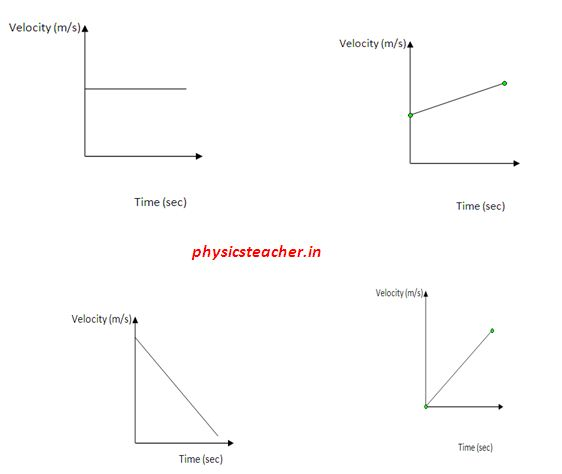# Velocity time graph

Velocity time graph is defined as a graphical representation of velocity of a body in motion with respect to time.

Generally velocity is assigned to the y axis and time is pointed on the x axis.

This graph type is also written as v-t graph or velocity-time graph.

The diagram below depicts few velocity time graphs, each depicting a specific type of linear motion.Here in this post we will explain a case study of velocity time graph.This graph will help you to understand different scenarios of a linear motion.

As we practically travel through linear motion, sometimes we have a uniform velocity, sometimes we just accelerate for a duration. And again we slow down through retardation.

The velocity-time graph presented here shows you all these kinds of scenarios. Hope you like this and it helps you to get the fundamentals clear.

## Velocity Time Graph – Case Study

From the above v-t graph, find out the distance travelled in each section A, B, C and D.

Also find out the total distance travelled.

### Explanation – section A

During this period velocity has changed from zero to 5 m/s .
And this change happened in a time duration of 10 seconds. That means the object in motion had an accelerated motion during this period.

From the equation of acceleration we get acceleration for section A = (v-u)/t = (5-0)/10 = 0.5 m/s2

[ t = time duration of a period = time at end of a period(t2) – time at the beginning of that period (t1) ]

The distance traversed in this period A = S1
= ut + ½ a t^2
S1 = 0 + ½ .0.5. 10^2=25 meter

### Explanation – section B

Here, you can see that the velocity didn’t change at all and stayed at 5 m/s for the duration of (30-10) sec = 20 seconds.

So for duration of 20 seconds of period B the object had a uniform motion with velocity 5 m/s.

Now the distance traversed in this period B = S2 = v t = 5.20 m=100 m

### Explanation – section C

In section C the object had again accelerated and changed its velocity from 5 m/s to 15m/s. And the time taken to make this velocity rise is = (40-30) s=10 seconds. we get the value of acceleration as =(v-u)/t =(15-5)/10 = 1 m/s^2

We can calculate the distance travelled during this period = S3.
S3 = ut + ½ a t^2 = 5.10 + 1/2 . 1.10^2 = 100 m

### Explanation – section D

In this section we find the object slowing down to zero from a velocity of 15 m/s. And for this it took a time period of = (50-40)sec=10 seconds again.

The retardation during this period is = (0-15)/10= – 1.5 m/s^2. (note the negative sign)

The distance travelled in this period is = S4
S4 = ut + ½ a t^2 = 15.10 – 1/2 . 1.5.10^2 = 75 m

### Velocity time graph – summary

Along the path the velocity has changed from zero to 5 m/s, then 15 m/s and then again back to zero.

The body has experienced different values of acceleration, even had uniform motion and a retardation.

The total distance travelled in 4 sections = (25+100+100+75)m = 300 meter.

Related study

#### Pulley in Physics – pulley tension problems#### Newton’s laws | Contact Forces between blocks | Application of Newton’s laws#### Elevator problems in Physics-5 Elevator Case Studies for you to solve#### Newton’s First Law of Motion doesn’t hold good for all frames of reference

Velocity time graph
Scroll to top
error: Content is protected !!